# Newton's laws of motion

"Laws of Motion" redirects here. For other uses, see Laws of motion.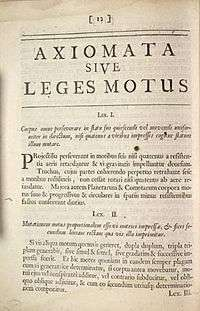Newton's First and Second laws, in Latin, from the original 1687 Principia Mathematica.

Newton's laws of motion are three physical laws that, together, laid the foundation for classical mechanics. They describe the relationship between a body and the forces acting upon it, and its motion in response to those forces. They have been expressed in several different ways, over nearly three centuries, and can be summarised as follows.

 First law: In an inertial reference frame, an object either remains at rest or continues to move at a constant velocity, unless acted upon by a net force. Second law: In an inertial reference frame, the vector sum of the forces F on an object is equal to the mass m of that object multiplied by the acceleration a of the object: F = ma. Third law: When one body exerts a force on a second body, the second body simultaneously exerts a force equal in magnitude and opposite in direction on the first body.

The three laws of motion were first compiled by Isaac Newton in his Philosophiæ Naturalis Principia Mathematica (Mathematical Principles of Natural Philosophy), first published in 1687. Newton used them to explain and investigate the motion of many physical objects and systems. For example, in the third volume of the text, Newton showed that these laws of motion, combined with his law of universal gravitation, explained Kepler's laws of planetary motion.

## Overview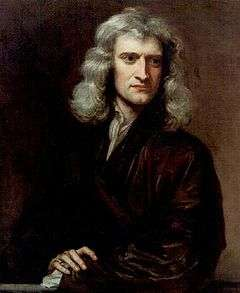Isaac Newton (1643–1727), the physicist who formulated the laws

Newton's laws are applied to objects which are idealised as single point masses, in the sense that the size and shape of the object's body are neglected to focus on its motion more easily. This can be done when the object is small compared to the distances involved in its analysis, or the deformation and rotation of the body are of no importance. In this way, even a planet can be idealised as a particle for analysis of its orbital motion around a star.

In their original form, Newton's laws of motion are not adequate to characterise the motion of rigid bodies and deformable bodies. Leonhard Euler in 1750 introduced a generalisation of Newton's laws of motion for rigid bodies called Euler's laws of motion, later applied as well for deformable bodies assumed as a continuum. If a body is represented as an assemblage of discrete particles, each governed by Newton's laws of motion, then Euler's laws can be derived from Newton's laws. Euler's laws can, however, be taken as axioms describing the laws of motion for extended bodies, independently of any particle structure.

Newton's laws hold only with respect to a certain set of frames of reference called Newtonian or inertial reference frames. Some authors interpret the first law as defining what an inertial reference frame is; from this point of view, the second law holds only when the observation is made from an inertial reference frame, and therefore the first law cannot be proved as a special case of the second. Other authors do treat the first law as a corollary of the second. The explicit concept of an inertial frame of reference was not developed until long after Newton's death.

In the given interpretation mass, acceleration, momentum, and (most importantly) force are assumed to be externally defined quantities. This is the most common, but not the only interpretation of the way one can consider the laws to be a definition of these quantities.

Newtonian mechanics has been superseded by special relativity, but it is still useful as an approximation when the speeds involved are much slower than the speed of light.

## Newton's first law

Main article: Inertia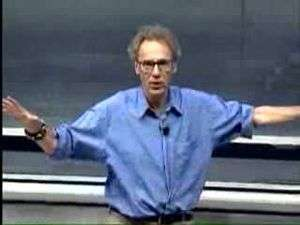Explanation of Newton's first law and reference frames. (MIT Course 8.01)

The first law states that if the net force (the vector sum of all forces acting on an object) is zero, then the velocity of the object is constant. Velocity is a vector quantity which expresses both the object's speed and the direction of its motion; therefore, the statement that the object's velocity is constant is a statement that both its speed and the direction of its motion are constant.

The first law can be stated mathematically when the mass is a non-zero constant, as,Consequently,

• An object that is at rest will stay at rest unless a force acts upon it.
• An object that is in motion will not change its velocity unless a force acts upon it.

This is known as uniform motion. An object continues to do whatever it happens to be doing unless a force is exerted upon it. If it is at rest, it continues in a state of rest (demonstrated when a tablecloth is skilfully whipped from under dishes on a tabletop and the dishes remain in their initial state of rest). If an object is moving, it continues to move without turning or changing its speed. This is evident in space probes that continually move in outer space. Changes in motion must be imposed against the tendency of an object to retain its state of motion. In the absence of net forces, a moving object tends to move along a straight line path indefinitely.

Newton placed the first law of motion to establish frames of reference for which the other laws are applicable. The first law of motion postulates the existence of at least one frame of reference called a Newtonian or inertial reference frame, relative to which the motion of a particle not subject to forces is a straight line at a constant speed. Newton's first law is often referred to as the law of inertia. Thus, a condition necessary for the uniform motion of a particle relative to an inertial reference frame is that the total net force acting on it is zero. In this sense, the first law can be restated as:

In every material universe, the motion of a particle in a preferential reference frame Φ is determined by the action of forces whose total vanished for all times when and only when the velocity of the particle is constant in Φ. That is, a particle initially at rest or in uniform motion in the preferential frame Φ continues in that state unless compelled by forces to change it.

Newton's first and second laws are valid only in an inertial reference frame. Any reference frame that is in uniform motion with respect to an inertial frame is also an inertial frame, i.e. Galilean invariance or the principle of Newtonian relativity.

## Newton's second law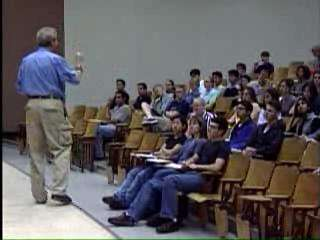Explanation of Newton's second law, using gravity as an example. (MIT OCW)

The second law states that the rate of change of momentum of a body, is directly proportional to the force applied and this change in momentum takes place in the direction of the applied force.The second law can also be stated in terms of an object's acceleration. Since Newton's second law is valid only for constant-mass systems, it can be taken outside the differentiation operator by the constant factor rule in differentiation. Thus,where F is the net force applied, m is the mass of the body, and a is the body's acceleration. Thus, the net force applied to a body produces a proportional acceleration. In other words, if a body is accelerating, then there is a force on it.

Consistent with the first law, the time derivative of the momentum is non-zero when the momentum changes direction, even if there is no change in its magnitude; such is the case with uniform circular motion. The relationship also implies the conservation of momentum: when the net force on the body is zero, the momentum of the body is constant. Any net force is equal to the rate of change of the momentum.

Any mass that is gained or lost by the system will cause a change in momentum that is not the result of an external force. A different equation is necessary for variable-mass systems (see below).

Newton's second law requires modification if the effects of special relativity are to be taken into account, because at high speeds the approximation that momentum is the product of rest mass and velocity is not accurate.

### Impulse

An impulse J occurs when a force F acts over an interval of time Δt, and it is given bySince force is the time derivative of momentum, it follows thatThis relation between impulse and momentum is closer to Newton's wording of the second law.

Impulse is a concept frequently used in the analysis of collisions and impacts.

### Variable-mass systems

Main article: Variable-mass system

Variable-mass systems, like a rocket burning fuel and ejecting spent gases, are not closed and cannot be directly treated by making mass a function of time in the second law; that is, the following formula is wrong:The falsehood of this formula can be seen by noting that it does not respect Galilean invariance: a variable-mass object with F = 0 in one frame will be seen to have F ≠ 0 in another frame. The correct equation of motion for a body whose mass m varies with time by either ejecting or accreting mass is obtained by applying the second law to the entire, constant-mass system consisting of the body and its ejected/accreted mass; the result iswhere u is the velocity of the escaping or incoming mass relative to the body. From this equation one can derive the equation of motion for a varying mass system, for example, the Tsiolkovsky rocket equation. Under some conventions, the quantity udm/dt on the left-hand side, which represents the advection of momentum, is defined as a force (the force exerted on the body by the changing mass, such as rocket exhaust) and is included in the quantity F. Then, by substituting the definition of acceleration, the equation becomes F = ma.

## Newton's third lawAn illustration of Newton's third law in which two skaters push against each other. The first skater on the left exerts a normal force N12 on the second skater directed towards the right, and the second skater exerts a normal force N21 on the first skater directed towards the left.
The magnitudes of both forces are equal, but they have opposite directions, as dictated by Newton's third law.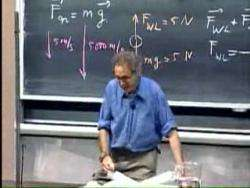A description of Newton's third law and contact forces

The third law states that all forces between two objects exist in equal magnitude and opposite direction: if one object A exerts a force FA on a second object B, then B simultaneously exerts a force FB on A, and the two forces are equal in magnitude and opposite in direction: FA = −FB. The third law means that all forces are interactions between different bodies, or different regions within one body, and thus that there is no such thing as a force that is not accompanied by an equal and opposite force. In some situations, the magnitude and direction of the forces are determined entirely by one of the two bodies, say Body A; the force exerted by Body A on Body B is called the "action", and the force exerted by Body B on Body A is called the "reaction". This law is sometimes referred to as the action-reaction law, with FA called the "action" and FB the "reaction". In other situations the magnitude and directions of the forces are determined jointly by both bodies and it isn't necessary to identify one force as the "action" and the other as the "reaction". The action and the reaction are simultaneous, and it does not matter which is called the action and which is called reaction; both forces are part of a single interaction, and neither force exists without the other.

The two forces in Newton's third law are of the same type (e.g., if the road exerts a forward frictional force on an accelerating car's tires, then it is also a frictional force that Newton's third law predicts for the tires pushing backward on the road).

From a conceptual standpoint, Newton's third law is seen when a person walks: they push against the floor, and the floor pushes against the person. Similarly, the tires of a car push against the road while the road pushes back on the tires—the tires and road simultaneously push against each other. In swimming, a person interacts with the water, pushing the water backward, while the water simultaneously pushes the person forward—both the person and the water push against each other. The reaction forces account for the motion in these examples. These forces depend on friction; a person or car on ice, for example, may be unable to exert the action force to produce the needed reaction force.

## History

### Newton's 1st Law

From the original Latin of Newton's Principia:

 “ Lex I: Corpus omne perseverare in statu suo quiescendi vel movendi uniformiter in directum, nisi quatenus a viribus impressis cogitur statum illum mutare. ”

 “ Law I: Every body persists in its state of being at rest or of moving uniformly straight forward, except insofar as it is compelled to change its state by force impressed. ”

The ancient Greek philosopher Aristotle had the view that all objects have a natural place in the universe: that heavy objects (such as rocks) wanted to be at rest on the Earth and that light objects like smoke wanted to be at rest in the sky and the stars wanted to remain in the heavens. He thought that a body was in its natural state when it was at rest, and for the body to move in a straight line at a constant speed an external agent was needed continually to propel it, otherwise it would stop moving. Galileo Galilei, however, realised that a force is necessary to change the velocity of a body, i.e., acceleration, but no force is needed to maintain its velocity. In other words, Galileo stated that, in the absence of a force, a moving object will continue moving. (The tendency of objects to resist changes in motion was what Johannes Kepler had called inertia.) This insight was refined by Newton, who made it into his first law, also known as the "law of inertia"—no force means no acceleration, and hence the body will maintain its velocity. As Newton's first law is a restatement of the law of inertia which Galileo had already described, Newton appropriately gave credit to Galileo.

The law of inertia apparently occurred to several different natural philosophers and scientists independently, including Thomas Hobbes in his Leviathan. The 17th century philosopher and mathematician René Descartes also formulated the law, although he did not perform any experiments to confirm it.

### Newton's 2nd Law

 “ Lex II: Mutationem motus proportionalem esse vi motrici impressae, et fieri secundum lineam rectam qua vis illa imprimitur. ”

This was translated quite closely in Motte's 1729 translation as:

 “ Law II: The alteration of motion is ever proportional to the motive force impress'd; and is made in the direction of the right line in which that force is impress'd. ”

According to modern ideas of how Newton was using his terminology, this is understood, in modern terms, as an equivalent of:

The change of momentum of a body is proportional to the impulse impressed on the body, and happens along the straight line on which that impulse is impressed.

This may be expressed by the formula F = p', where p' is the time derivative of the momentum p. This equation can be seen clearly in the Wren Library of Trinity College, Cambridge, in a glass case in which Newton's manuscript is open to the relevant page.

Motte's 1729 translation of Newton's Latin continued with Newton's commentary on the second law of motion, reading:

If a force generates a motion, a double force will generate double the motion, a triple force triple the motion, whether that force be impressed altogether and at once, or gradually and successively. And this motion (being always directed the same way with the generating force), if the body moved before, is added to or subtracted from the former motion, according as they directly conspire with or are directly contrary to each other; or obliquely joined, when they are oblique, so as to produce a new motion compounded from the determination of both.

The sense or senses in which Newton used his terminology, and how he understood the second law and intended it to be understood, have been extensively discussed by historians of science, along with the relations between Newton's formulation and modern formulations.

### Newton's 3rd Law

 “ Lex III: Actioni contrariam semper et æqualem esse reactionem: sive corporum duorum actiones in se mutuo semper esse æquales et in partes contrarias dirigi. ”

 “ Law III: To every action there is always opposed an equal reaction: or the mutual actions of two bodies upon each other are always equal, and directed to contrary parts. ”

Newton's Scholium (explanatory comment) to this law:

Whatever draws or presses another is as much drawn or pressed by that other. If you press a stone with your finger, the finger is also pressed by the stone. If a horse draws a stone tied to a rope, the horse (if I may so say) will be equally drawn back towards the stone: for the distended rope, by the same endeavour to relax or unbend itself, will draw the horse as much towards the stone, as it does the stone towards the horse, and will obstruct the progress of the one as much as it advances that of the other. If a body impinges upon another, and by its force changes the motion of the other, that body also (because of the equality of the mutual pressure) will undergo an equal change, in its own motion, toward the contrary part. The changes made by these actions are equal, not in the velocities but in the motions of the bodies; that is to say, if the bodies are not hindered by any other impediments. For, as the motions are equally changed, the changes of the velocities made toward contrary parts are reciprocally proportional to the bodies. This law takes place also in attractions, as will be proved in the next scholium.

In the above, as usual, motion is Newton's name for momentum, hence his careful distinction between motion and velocity.

Newton used the third law to derive the law of conservation of momentum; from a deeper perspective, however, conservation of momentum is the more fundamental idea (derived via Noether's theorem from Galilean invariance), and holds in cases where Newton's third law appears to fail, for instance when force fields as well as particles carry momentum, and in quantum mechanics.

## Importance and range of validity

Newton's laws were verified by experiment and observation for over 200 years, and they are excellent approximations at the scales and speeds of everyday life. Newton's laws of motion, together with his law of universal gravitation and the mathematical techniques of calculus, provided for the first time a unified quantitative explanation for a wide range of physical phenomena.

These three laws hold to a good approximation for macroscopic objects under everyday conditions. However, Newton's laws (combined with universal gravitation and classical electrodynamics) are inappropriate for use in certain circumstances, most notably at very small scales, very high speeds (in special relativity, the Lorentz factor must be included in the expression for momentum along with the rest mass and velocity) or very strong gravitational fields. Therefore, the laws cannot be used to explain phenomena such as conduction of electricity in a semiconductor, optical properties of substances, errors in non-relativistically corrected GPS systems and superconductivity. Explanation of these phenomena requires more sophisticated physical theories, including general relativity and quantum field theory.

In quantum mechanics, concepts such as force, momentum, and position are defined by linear operators that operate on the quantum state; at speeds that are much lower than the speed of light, Newton's laws are just as exact for these operators as they are for classical objects. At speeds comparable to the speed of light, the second law holds in the original form F = dp/dt, where F and p are four-vectors.

## Relationship to the conservation laws

In modern physics, the laws of conservation of momentum, energy, and angular momentum are of more general validity than Newton's laws, since they apply to both light and matter, and to both classical and non-classical physics.

This can be stated simply, "Momentum, energy and angular momentum cannot be created or destroyed."

Because force is the time derivative of momentum, the concept of force is redundant and subordinate to the conservation of momentum, and is not used in fundamental theories (e.g., quantum mechanics, quantum electrodynamics, general relativity, etc.). The standard model explains in detail how the three fundamental forces known as gauge forces originate out of exchange by virtual particles. Other forces, such as gravity and fermionic degeneracy pressure, also arise from the momentum conservation. Indeed, the conservation of 4-momentum in inertial motion via curved space-time results in what we call gravitational force in general relativity theory. The application of the space derivative (which is a momentum operator in quantum mechanics) to the overlapping wave functions of a pair of fermions (particles with half-integer spin) results in shifts of maxima of compound wavefunction away from each other, which is observable as the "repulsion" of the fermions.

Newton stated the third law within a world-view that assumed instantaneous action at a distance between material particles. However, he was prepared for philosophical criticism of this action at a distance, and it was in this context that he stated the famous phrase "I feign no hypotheses". In modern physics, action at a distance has been completely eliminated, except for subtle effects involving quantum entanglement. However, in modern engineering, in all practical applications involving the motion of vehicles and satellites, the concept of action at a distance is used extensively.

The discovery of the second law of thermodynamics by Carnot in the 19th century showed that every physical quantity is not conserved over time, thus disproving the validity of inducing the opposite metaphysical view from Newton's laws. Hence, a "steady-state" worldview based solely on Newton's laws and the conservation laws does not take entropy into account.Wikimedia Commons has media related to Newton's laws of motion.

## References and notes

1. For explanations of Newton's laws of motion by Newton in the early 18th century, by the physicist William Thomson (Lord Kelvin) in the mid-19th century, and by a modern text of the early 21st century, see:-
2. Browne, Michael E. (July 1999). Schaum's outline of theory and problems of physics for engineering and science (Series: Schaum's Outline Series). McGraw-Hill Companies. p. 58. ISBN 978-0-07-008498-8.
3. Holzner, Steven (December 2005). Physics for Dummies. Wiley, John & Sons, Incorporated. p. 64. ISBN 978-0-7645-5433-9.
4. See the Principia on line at Andrew Motte Translation
5. Andrew Motte translation of Newton's Principia (1687) Axioms or Laws of Motion
6. [...]while Newton had used the word 'body' vaguely and in at least three different meanings, Euler realized that the statements of Newton are generally correct only when applied to masses concentrated at isolated points;Truesdell, Clifford A.; Becchi, Antonio; Benvenuto, Edoardo (2003). Essays on the history of mechanics: in memory of Clifford Ambrose Truesdell and Edoardo Benvenuto. New York: Birkhäuser. p. 207. ISBN 3-7643-1476-1.
7. Lubliner, Jacob (2008). Plasticity Theory (Revised Edition) (PDF). Dover Publications. ISBN 0-486-46290-0.
8. Galili, I.; Tseitlin, M. (2003). "Newton's First Law: Text, Translations, Interpretations and Physics Education". Science & Education. 12 (1): 45–73. Bibcode:2003Sc&Ed..12...45G. doi:10.1023/A:1022632600805.
9. Benjamin Crowell. "4. Force and Motion". Newtonian Physics. ISBN 0-9704670-1-X.
10. In making a modern adjustment of the second law for (some of) the effects of relativity, m would be treated as the relativistic mass, producing the relativistic expression for momentum, and the third law might be modified if possible to allow for the finite signal propagation speed between distant interacting particles.
11. Walter Lewin (20 September 1999). Newton's First, Second, and Third Laws. MIT Course 8.01: Classical Mechanics, Lecture 6. (ogg) (videotape). Cambridge, MA USA: MIT OCW. Event occurs at 0:00–6:53. Retrieved 23 December 2010.
12. NMJ Woodhouse (2003). Special relativity. London/Berlin: Springer. p. 6. ISBN 1-85233-426-6.
13. Beatty, Millard F. (2006). Principles of engineering mechanics Volume 2 of Principles of Engineering Mechanics: Dynamics-The Analysis of Motion,. Springer. p. 24. ISBN 0-387-23704-6.
14. Thornton, Marion (2004). Classical dynamics of particles and systems (5th ed.). Brooks/Cole. p. 53. ISBN 0-534-40896-6.
15. Lewin, Newton’s First, Second, and Third Laws, Lecture 6. (6:53–11:06)
16. Plastino, Angel R.; Muzzio, Juan C. (1992). "On the use and abuse of Newton's second law for variable mass problems". Celestial Mechanics and Dynamical Astronomy. Netherlands: Kluwer Academic Publishers. 53 (3): 227–232. Bibcode:1992CeMDA..53..227P. doi:10.1007/BF00052611. ISSN 0923-2958. "We may conclude emphasizing that Newton's second law is valid for constant mass only. When the mass varies due to accretion or ablation, [an alternate equation explicitly accounting for the changing mass] should be used."
17. Halliday; Resnick. Physics. 1. p. 199. ISBN 0-471-03710-9. It is important to note that we cannot derive a general expression for Newton's second law for variable mass systems by treating the mass in F = dP/dt = d(Mv) as a variable. [...] We can use F = dP/dt to analyze variable mass systems only if we apply it to an entire system of constant mass having parts among which there is an interchange of mass. [Emphasis as in the original]
18. Kleppner, Daniel; Robert Kolenkow (1973). An Introduction to Mechanics. McGraw-Hill. pp. 133–134. ISBN 0-07-035048-5. Recall that F = dP/dt was established for a system composed of a certain set of particles[. ... I]t is essential to deal with the same set of particles throughout the time interval[. ...] Consequently, the mass of the system can not change during the time of interest.
19. Hannah, J, Hillier, M J, Applied Mechanics, p221, Pitman Paperbacks, 1971
20. Raymond A. Serway; Jerry S. Faughn (2006). College Physics. Pacific Grove CA: Thompson-Brooks/Cole. p. 161. ISBN 0-534-99724-4.
21. I Bernard Cohen (Peter M. Harman & Alan E. Shapiro, Eds) (2002). The investigation of difficult things: essays on Newton and the history of the exact sciences in honour of D.T. Whiteside. Cambridge UK: Cambridge University Press. p. 353. ISBN 0-521-89266-X.
22. WJ Stronge (2004). Impact mechanics. Cambridge UK: Cambridge University Press. p. 12 ff. ISBN 0-521-60289-0.
23. Lewin, Newton’s First, Second, and Third Laws, Lecture 6. (14:11–16:00)
24. Resnick; Halliday; Krane (1992). Physics, Volume 1 (4th ed.). p. 83.
25. C Hellingman (1992). "Newton's third law revisited". Phys. Educ. 27 (2): 112–115. Bibcode:1992PhyEd..27..112H. doi:10.1088/0031-9120/27/2/011. Quoting Newton in the Principia: It is not one action by which the Sun attracts Jupiter, and another by which Jupiter attracts the Sun; but it is one action by which the Sun and Jupiter mutually endeavour to come nearer together.
26. Resnick & Halliday (1977). Physics (Third ed.). John Wiley & Sons. pp. 78–79. Any single force is only one aspect of a mutual interaction between two bodies.
27. Hewitt (2006), p. 75
28. Isaac Newton, The Principia, A new translation by I.B. Cohen and A. Whitman, University of California press, Berkeley 1999.
29. Thomas Hobbes wrote in Leviathan:
That when a thing lies still, unless somewhat else stir it, it will lie still forever, is a truth that no man doubts. But [the proposition] that when a thing is in motion it will eternally be in motion unless somewhat else stay it, though the reason be the same (namely that nothing can change itself), is not so easily assented to. For men measure not only other men but all other things by themselves. And because they find themselves subject after motion to pain and lassitude, [they] think every thing else grows weary of motion and seeks repose of its own accord, little considering whether it be not some other motion wherein that desire of rest they find in themselves, consists.
30. Cohen, I. B. (1995). Science and the Founding Fathers: Science in the Political Thought of Jefferson, Franklin, Adams and Madison. New York: W.W. Norton. p. 117. ISBN 978-0393315103.
31. Cohen, I. B. (1980). The Newtonian Revolution: With Illustrations of the Transformation of Scientific Ideas. Cambridge, England: Cambridge University Press. p. 183-4. ISBN 978-0521273800.
32. According to Maxwell in Matter and Motion, Newton meant by motion "the quantity of matter moved as well as the rate at which it travels" and by impressed force he meant "the time during which the force acts as well as the intensity of the force". See Harman and Shapiro, cited below.
33. See for example (1) I Bernard Cohen, "Newton's Second Law and the Concept of Force in the Principia", in "The Annus Mirabilis of Sir Isaac Newton 1666–1966" (Cambridge, Massachusetts: The MIT Press, 1967), pages 143–185; (2) Stuart Pierson, "'Corpore cadente. . .': Historians Discuss Newton’s Second Law", Perspectives on Science, 1 (1993), pages 627–658; and (3) Bruce Pourciau, "Newton's Interpretation of Newton's Second Law", Archive for History of Exact Sciences, vol.60 (2006), pages 157–207; also an online discussion by G E Smith, in 5. Newton's Laws of Motion, s.5 of "Newton's Philosophiae Naturalis Principia Mathematica" in (online) Stanford Encyclopedia of Philosophy, 2007.
34. This translation of the third law and the commentary following it can be found in the "Principia" on page 20 of volume 1 of the 1729 translation.
35. Newton, Principia, Corollary III to the laws of motion

## Further reading and works cited

• Crowell, Benjamin (2011), Light and Matter (2011, Light and Matter), especially at Section 4.2, Newton's First Law, Section 4.3, Newton's Second Law, and Section 5.1, Newton's Third Law.
• Feynman, R. P.; Leighton, R. B.; Sands, M. (2005). The Feynman Lectures on Physics. Vol. 1 (2nd ed.). Pearson/Addison-Wesley. ISBN 0-8053-9049-9.
• Fowles, G. R.; Cassiday, G. L. (1999). Analytical Mechanics (6th ed.). Saunders College Publishing. ISBN 0-03-022317-2.
• Likins, Peter W. (1973). Elements of Engineering Mechanics. McGraw-Hill Book Company. ISBN 0-07-037852-5.
• Marion, Jerry; Thornton, Stephen (1995). Classical Dynamics of Particles and Systems. Harcourt College Publishers. ISBN 0-03-097302-3.
• NMJ Woodhouse (2003). Special Relativity. London/Berlin: Springer. p. 6. ISBN 1-85233-426-6.
Historical